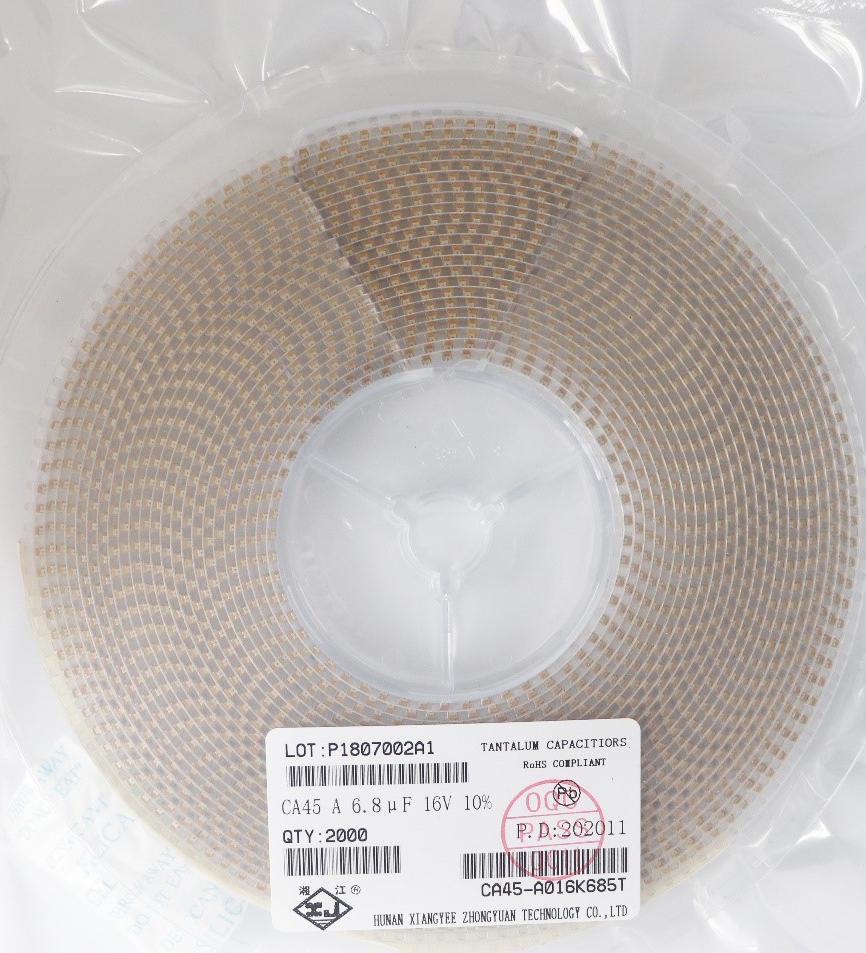# 电容的国际单位及换算方式

1F=1000mF
1mF=1000μF
1μF=1000nF
1nF=1000pF
105=10x105pF=1000,000pF=1000nF=1μF
104=100nF=0.1μF
103=0.01μF=10nF=10,000pF
102=0.001μF=1nF=1000pF
224=22*104pF=0.22μF?在实际应用上，法拉这一单位太大了，我们一般不用。电解电容的容量是非常大的，我们通常用的是nF(钠法)、pF(皮法)，而钽电容相对而言在同等体积下能做到的容量比较大，因此单位通常为μF（微法）。